##### Pre-Calculus: 1001 Practice Problems For Dummies (+ Free Online Practice)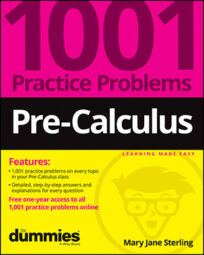Conic sections can be described or illustrated with exactly what their name suggests: cones. Imagine an orange cone in the street, steering you in the right direction. Then picture some clever highway engineer placing one cone on top of the other, tip to tip. That engineer is trying to demonstrate how you can create conic sections.

If you come along and slice one of those cones parallel to the ground, the cut edges form a circle. Slice the cone on an angle, and you have an ellipse. Slice the cone parallel to one of the sides, and you have a parabola. And, finally, slice through both cones together, perpendicular to the ground, and you have a hyperbola.

If these descriptions just don’t work for you, the practice problems should do the trick.

You’ll work on conic sections in the following ways:

• Recognizing which conic you have from the general equation

• Finding the centers of circles and ellipses

• Determining the foci of circles, ellipses, and parabolas

• Using the directrix of a parabola to complete the sketch

• Writing the equations of a hyperbola’s asymptotes

• Changing basic conic section equations from parametric to rectangular

When working with conic sections, some challenges will include the following:

• Determining the major axis of an ellipse

• Sketching the graph of a parabola in the correct direction

• Using the asymptotes of a hyperbola correctly in a graph

• Finding the square root in the equation of a circle when finding the radius

## Practice problems

1. Name the conic and its center.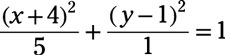The standard form for the equation of an ellipse with center (h, k) is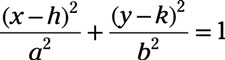The given equation is already in this form, so you can identify the center coordinates by looking at the values substituted for h and k.

2. Write the equation of the circle described. Then graph the circle: center: (4, 3); radius: 5

Answer: (x – 4)2 + (y – 3)2 = 25

The standard equation of a circle with radius (h, k) and radius r is (xh)2 + (yk)2 = r2. Substitute the given point (4, 3) for the (h, k) and square the 5: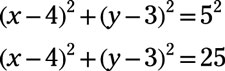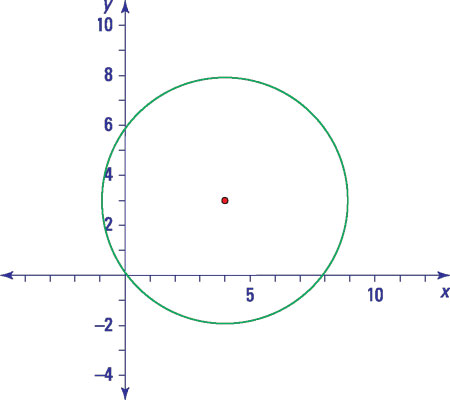Credit: Illustration by Thomson Digital